# Samacheer Kalvi 6th Maths Solutions Term 3 Chapter 4 Geometry Additional Questions## Tamilnadu Samacheer Kalvi 6th Maths Solutions Term 3 Chapter 4 Geometry Additional Questions

Question 1.
List any three letters having same mirror image as the object or letters?
Solution:
A, O and T

Question 2.
In the word SYMMETRY which letters do not have identical mirror images?
Solution:
S, E and RQuestion 3.
Is Indian National flag symmetrical ?
Solution:
Yes, Horizontal

Question 4.
In the word ‘FIGURE’ mention the symmetrical letters.
Solution:
I, U and E

Question 5.
Are the number of symmetrical lines equal in rectangle and square?
Solution:
No, not equal.

Question 6.
Find the number of lines of symmetry in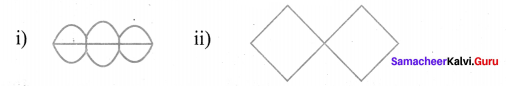i) The number of lines of symmetry
ii) The number of lines of symmetry
Solution:
i) The number of lines of symmetry for this figure are 2.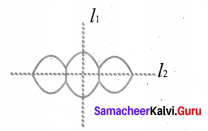ii) The number of lines of symmetry for this figure are 2.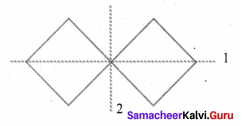Question 7.
Give the reflection of the letter M as shown in the figure.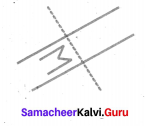Solution: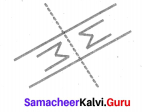Question 8.
Find the number of lines of symmetry of the following.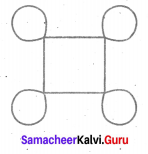Solution: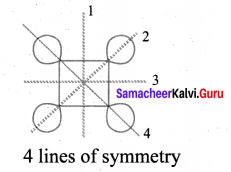Question 9.
Are human faces symmetrical ?
Solution:
Yes

Question 10.
List some symmetrical objects from your home?
Solution:
Wall clock, dining table top, bucket, photo frame etc.